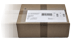# TI-30X Pro MathPrint

sales promotion
CHF 25.50CHF 29.00When you order this item your purchase will be despatched free or charge
Availability
Available from stock  Delivery within 1-3 working days!
Manufacturer
Texas Instruments
Guarantee
36 Months
GTIN
03243480105927
Dimensions
80 x 184 x 16 mm
Weight
130 g
Directions for Use
German, French, Italian, English

#### Items delivered

• TI-30X Pro MathPrint calculator
• Protective cover
• Batterie (2 x CR2032)

Also available: TI-SmartView MathPrint Emulator Software.
The Emulator Software offers a greater range of functions than the TI-30X Pro MathPrint school calculator, providing additional options for preparing and giving lessons. Show the class how the school calculator works. There are two different view options to choose from: TI-30X Pro MathPrint and TI-30X Plus MathPrint. Or use previously prepared notes in the lesson. Ideal in combination with interactive whiteboards.
The new school scientific calculator TI-30X Pro MathPrint by Texas Instruments is so technically advanced that it is recommended for use in secondary schools and higher education.
• High-resolution display with up to four lines of 16 characters guarantees easier readability and enables e.g. the display of two functions in the value table
• "MathPrint": Input and output in mathematical notation (as in the maths book)
• NEW: Operating system display ensures greater exam security
• Equation solver (numerical equation, polynomial and system linear equations)
• Numerical differentiation and integration of real functions
• Vector and matrix calculations
• A wide range of functions for arithmetic, algebra, geometry, trigonometry, stochastics and analysis
• Fractions with a horizontal fraction bar and fraction function calculations (reduction, conversion of fractions/decimals/percentages; change between improper fractions and mixed numbers)
• Converts at the touch of a button: fractions, square roots and Pi into decimals and vice versa
• Prime factorisation, GCD and LCM functions
• Easy calculation with variables and function values
• Calculates partial sum and partial product
• Increased functionality for statistical data analysis
• Enter and store value tables and assign any desired functions
• Basic data/list editor with three lists and list formulae
• One- and two-variable statistics with continuous input autosave for statistical variables.
• Stochastic functions: calculates n!, means and standard deviations plus probabilities, in particular binomial and normal distributions
• Broad range of regression models (linear, non-linear and NEW: natural exponential functions (e^x))
• Measures of location and dispersion, also the median and quartiles
• Calculating with complex numbers
• Summation of any terms
• Calculates with and converts between binary, octal, decimal and hexadecimal numbers (BIN/OCT/DEC/HEX)
• Metric conversions and physical constants
• Random generator for numbers and integers
• The Equation Recall function calls up previous inputs, saved values and individual statistics from the memory.
• Continuous autosave: no entry gets lost
• Memory for seven variables (x,y,z,t,a,b,c)
• Battery operation backed up by solar cells to prolong battery life
Accessories

^ top